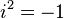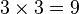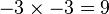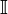# Imaginary number facts for kids

Kids Encyclopedia Facts

Imaginary numbers are numbers that are made from combining a real number with the imaginary unit, called i, where i is defined as$i^2=-1$. They are defined separately from the negative real numbers in that they are a square root of a negative real number (instead of a positive real number). This is not possible with real numbers, as there is no real number that will multiply by itself to get a negative number (e.g.,$3 \times 3 = 9$ and$-3 \times -3 = 9$). The set of imaginary numbers are sometimes denoted by the letter$\mathbb{I}$.

One way of thinking about imaginary numbers is to say that they are to negative numbers what negative numbers are to positive numbers. If we say "go east by -1 mile", it is the same as if we had said "go west by 1 mile". If we say "go east by i miles", it means the same thing as if we had said "go north by 1 mile". Similarly, if we say "go east by -i mile", it means the same as if we had said "go south by 1 mile".

Adding is easy too. If we say "go east by 1 + i miles" it means the same as if we had said "go east by one mile and north by one mile".

Multiplying two imaginary numbers is a lot like multiplying a positive number with a negative number. If we say "go east by 2 × -3 miles", it means "rotate all of the way around (so that you are now facing west) and go 2×3 = 6 miles". Imaginary numbers work the same, except that you can rotate part way. If we say go "east by 2×3i miles", it means the same as if we had said "rotate until you are facing north, and then go 2×3 = 6 miles"

Subtractions such as 5 - 9 used to be impossible until negative numbers were invented, just as taking the square root of a negative number used to be impossible until imaginary numbers were invented. The square root of 9 is 3, but the square root of −9 is not −3. This is because −3 x −3 = +9, not −9. For a long time, it seemed as though there was no answer to the square root of −9.

This is why mathematicians invented the imaginary number, i, and said that it is the main square root of −1. The square root of −1 is not a real number, so this definition creates a new type of number, just like fractions create numbers like 2/3 that are not counting numbers like 4 or 10, and negative numbers create numbers that are less than 0. Sometimes, mathematicians seem rather comfortable using a number that is so unusual, but the name imaginary should not fool you because i is as valid a number as 3 or 145,379.

Many branches of science and engineering have found uses for this number. For example. electrical engineers need i to understand how an electric circuit will work when they are designing it (electrical engineers use j instead of i to avoid confusion with the symbol for the current). As another example. certain branches of physics such as quantum physics and high energy physics use i as often as they use any other regular number. Many of the equations in the world simply cannot be solved without i.

Imaginary numbers can be mixed with numbers we are more familiar with. For example, a real number such as 2 can be added to an imaginary number such as 3i to create 2+3i. These kinds of mixed numbers are known as complex numbers.

## Related pagesImaginary number Facts for Kids. Kiddle Encyclopedia.